Updating search results...

# 11 Results

View
Selected filters:
• fair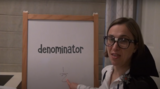Unrestricted Use
CC BY
Rating
0.0 stars

This lesson is Day 10 in a series of 12 lessons around fraction equivalences and comparisons.

This video lesson serves as a review of equpartitioning in order to determine the fractional part of an area or length. This is a necessary foundational skill for comparing and finding equivalent fractions.

Subject:
Mathematics
Material Type:
Activity/Lab
Interactive
Lesson
Simulation
Author:
Dawne Coker
06/25/2020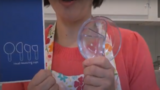Unrestricted Use
CC BY
Rating
0.0 stars

This lesson is Day 4 in a series of 12 lessons around fraction equivalences and comparisons.

This video lesson looks at how we use fractions in the kitchen. Students are challenged to use circle models to find equivalent fractions for a recipe. Students may use virtual circe models from the Toy Theater (https://toytheater.com/fraction-circles/).

Subject:
Mathematics
Material Type:
Activity/Lab
Interactive
Lesson
Simulation
Author:
Dawne Coker
06/25/2020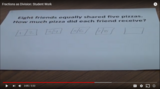Unrestricted Use
CC BY
Rating
0.0 stars

This video shows two different student-generated strategies for solving fair share problems leading to a fraction less than 1. This standard relates to Common Core standard 5.NF.3, and North Carolina standard NC.5.NF.3.

The end of this video helps teachers see how standard NF.3 can be introduced prior to NF.1 (addition/subraction of fractions with unlike denominators).

Subject:
Mathematics
Material Type:
Diagram/Illustration
Reference Material
Teaching/Learning Strategy
Author:
Dawne Coker
06/26/2020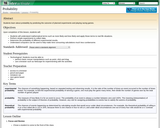Rating
0.0 stars

This lesson is designed to develop students' understanding of probability in real life situations. Students will also be introduced to running experiments, experimental probability, and theoretical probability. This lesson provides links to discussions and activities related to probability as well as suggested ways to integrate them into the lesson. Finally, the lesson provides links to follow-up lessons designed for use in succession with the current one.

Subject:
Mathematics
Material Type:
Lesson Plan
Provider:
CPALMS
02/26/2019Unrestricted Use
CC BY
Rating
0.0 stars

This resource is from Tools4NCTeachers.A Common Formative Assessment (CFA) provides a snapshot of current student understanding. It serves as a checkpoint, and may be used to guide future whole and small group instruction. This CFA addresses 2nd grade, Cluster 9&nbsp;standards. &nbsp;Answer key is provided.

Subject:
Mathematics
Material Type:
Assessment
Formative Assessment
Author:
DAWNE COKER
06/16/2020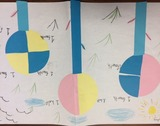Unrestricted Use
CC BY
Rating
0.0 stars

This resource is from Tools4NCTeachers.In this lesson, students partition circles into halves, thirds and fourths as they create fraction flowers.&nbsp; Student recording sheets and sample pictures of student work are included within the lesson.&nbsp; Links to virtual tools and extension ideas are also provided.&nbsp;&nbsp;Remix this lesson to include pictures of your students&#39; work!

Subject:
Mathematics
Material Type:
Activity/Lab
Lesson
Lesson Plan
Author:
DAWNE COKER
06/24/2020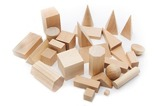Unrestricted Use
CC BY
Rating
0.0 stars

This resource is from Tools4NCTeachers.Students need distributed practice&nbsp;of newly-learned skills to develop fluency, build proficiency, and deepen understanding.&nbsp;This file contains directions for activities which may be used as Cluster 6 games or math stations.

Subject:
Mathematics
Material Type:
Activity/Lab
Game
Author:
DAWNE COKER
06/21/2020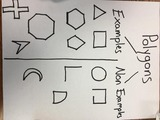Unrestricted Use
CC BY
Rating
0.0 stars

This resource is from Tools4NCTeachers. This file contains sample anchor charts to create with students. Anchor charts are to be made with students, then displayed as a classroom reference. Parents may also benefit from seeing anchor charts, as they provide a snapshot into the math class.Remix this resource to include pictures of your own anchor charts.

Subject:
Mathematics
Material Type:
Activity/Lab
Diagram/Illustration
Reference Material
Author:
DAWNE COKER
06/21/2020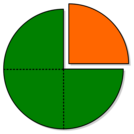Unrestricted Use
CC BY
Rating
0.0 stars

This resource is from Tools4NCTeachers.&nbsp;&nbsp;In this lesson students are introduced to the vocabulary associated with partitioning shapes into equal shares. Students will also explore shapes that are partitioned into halves, thirds, and fourths. Student recording sheets and pictures of anchor charts are included within this lesson.&nbsp;

Subject:
Mathematics
Material Type:
Activity/Lab
Lesson
Lesson Plan
Author:
DAWNE COKER
06/24/2020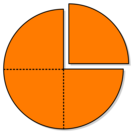Unrestricted Use
CC BY
Rating
0.0 stars

This resource is from Tools4NCTeachers.&nbsp;In this lesson, students explore partitioning shapes into halves and fourths.&nbsp; The lesson could be taught consecutively in one math block or extended over multiple days depending on students&rsquo; understanding of the concept of halves and fourths.

Subject:
Mathematics
Material Type:
Activity/Lab
Lesson
Lesson Plan
Author:
DAWNE COKER
06/12/2020Unrestricted Use
CC BY
Rating
0.0 stars

This resource is from Tools4NCTeachers.&nbsp;&nbsp;In this lesson, students will determine ways to partition a sandwich into two equal parts and determine if the halves are the same size.&nbsp; Then students will work with partners to determine different ways to partition a sandwich into fourths and prove that the fourths are equal shares. Sample student work and recording sheets are provided within this lesson.Remix this lesson to share extension activities and related math centers.

Subject:
Mathematics
Material Type:
Activity/Lab
Lesson
Lesson Plan
Author:
DAWNE COKER